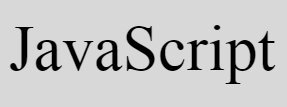# JavaScript Program to Check Prime Number

« Previous Program Next Program »

In this article, you will learn and get code to check whether the number entered by user is a prime number or not in JavaScript. Here are the list of programs you will go through:

• Check Prime Number or Not. This program does not allows user to enter the data
• Check whether a number entered by user is a Prime number or not. This program allows user to enter the number

### What is a Prime Number ?

A Prime number is a number that can only be divided exactly by 1 and the number itself. For example, 2, 3, 5, 7, 13, 17 etc.

As you can clearly see that, the number 17 can only be divided by 1 and 17. Similarly, 13 can only be divided by 1 and 13.

## Check Prime Number in JavaScript

Here is the simplest JavaScript program to check whether a number is a prime number or not. This program does not takes input from user.

```<!doctype html>
<html>
<body>
<script>
var num, i, chk=0;
num=19;
for(i=2; i<num; i++)
{
if(num%2==0)
{
chk++;
break;
}
}
if(chk==0)
document.write(num + " is a Prime Number");
else
document.write(num + " is not a Prime Number");
</script>
</body>
</html>```

Save this code in a file with .html extension. Open the file in a web browser. Here is the output produced:

Now change the value of num with 20. That is, initialize 20 to num. Save the code and open it again, or refresh the browser. Here is the output you will see:

Note - The document.write() method writes data into an HTML output.

### Get Number Input from User

Here is another program that asks from user to enter the number, to check and print whether the number entered by user is a prime number or not.

```<!doctype html>
<html>
<script>
var num, i, chk, temp;
function checkPrime()
{
num = parseInt(document.getElementById("num").value);
if(num)
{
chk=0;
temp = document.getElementById("resPara");
temp.style.display = "block";
for(i=2; i<num; i++)
{
if(num%2==0)
{
chk++;
break;
}
}
if(chk==0)
document.getElementById("res").innerHTML = "a Prime";
else
document.getElementById("res").innerHTML = "not a Prime";
}
}
</script>
<body>

<p>Enter the Number: <input id="num"><button onclick="checkPrime()">Check</button></p>
<p id="resPara" style="display:none;">It is <span id="res"></span> Number</p>

</body>
</html>```

Here is its sample output:

Now supply any number say 37 and click on Check button to check and print a message that told whether it is a prime number or not as shown in the snapshot given below:

The following code:

`style="display:none;"`

is a CSS code, hides an HTML element where it is present. Because it is present in a p (paragraph) tag whose id is resPara, therefore this paragraph gets hidden initially

When user clicks on the button, Check, a function named checkPrime() gets called. The following JavaScript code:

`num = parseInt(document.getElementById("num").value);`

states that, an int (integer) value of an HTML element whose id is num gets initialized to num variable. If num holds any value (instead of nothing or empty), the condition of if, num evaluates to be true, therefore program flow goes inside the loop.

The following JavaScript code/statement:

`temp.style.display = "block";`

states that, an HTML element whose id is stored in temp variable gets visible after executing this code. And the following code:

`document.getElementById("res").innerHTML = "a Prime";`

states that, the string value a Prime gets written to an HTML element whose id is res

#### Live Output of Previous Program

Here is the live output of previous program of JavaScript that asks from user to enter a number and checks whether it is a prime number or not.

Enter the Number:

JavaScript Online Test

« Previous Program Next Program »